# New Simplified Chemistry Class 7 ICSE Solutions – Language of Chemistry

## New Simplified Chemistry Class 7 ICSE Solutions – Language of Chemistry

Points to Remember:

1. A chemical reaction involves the transformation of original substance into an altogether new substance(s).
2. A chemical reaction can be represented with the help of the symbols or the formulae of the elements and the compounds taking part in that reaction. This gives a chemical equation.
3. Certain necessary conditions for a chemical reaction to happen are — close contact, solution form, heat, light and catalyst.
4. Characteristics of chemical reactions are — change of colour, evolution of a gas, formation of a precipitate, change of state, change of smell and evolution/absorption of heat.
5. A complete chemical equation symbolically represents the reactants, products and their physical states.
6. The substances that react with each other are called reactants and they are represented on the left hand side of the equation. The substances that are formed as a result of the reaction are called products. They are represented on the right hand side of the equation.
7. A chemical equation needs to be balanced to make it follow the law of the conservation of mass.
8. The law of conservation of mass states that mass can be neither created nor destroyed, it can only be transformed from one form to another.
9. A chemical equation gives both qualitative and quantitative information about the reactants and products.

Chemical Equations – Writing word equations Writing Word Equations
Write word equation for the following molecular equations:
Question 1.
CuCO3
CuO + CO2 [g]
Word equation:
State the characteristics or indications occurring in the above reaction.
Copper carbonate → Copper oxide + carbon dioxide
Indication –
The colour of Copper Carbonate (CuCO3) i.e. green changes to Copper oxide (CuO) i.e. Black

Question 2.word equation:
State which characteristic does [g] in the above reaction indicate.
The word equation ofIndication –
Evolution of gas i.e. Carbon dioxide.

Question 3.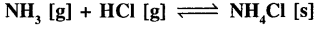Word equation:
State which characteristic is observed in the above reaction.
Give an-example of a similar above characteristic, seen between two other gaseous reactants.Indication –
Change of state from gas to solid.
Other example of change of state are:

1. 2H2 (g) + O2 (g) → 2H2O (l)
2. C (s) + 2S (s) → CS2 (l)

Question 4.
Na2SO3 + 2HCl [dil.]2NaCl + H2O + S02 [g]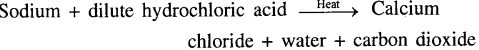Question 5.
FeSO4 + 2NaOH Na2SO4 + Fe(OH)2

Word equation:
State why [↓] indication is seen after Fe(OH)2 and not after Na2SO4
Iron (II) Sulphate + Sodium hydroxide → Sodium sulphate + Iron (II) hydroxide ↓
The precipitate formed in a chemical equation is shown with an arrow pointing downwards [↓].
Hence, Fe(OH)2 is the precipitate formed but not Na2SO4.

Question 6.Word equation:
State why [+ Δ] indication is seen after NH3.
State what 200 atmospheres – indicates.
State the function of Fe in the above reaction.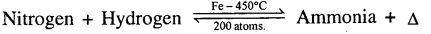1. + Δ at the end of the reaction represents heat evolved.
2. NH4 (Ammonia) decomposes in the presence of 200 atmospheric pressure.
3. Fe(Iron) act as catalyst in the reaction.

Question 7.Word equation:
State which form of energy is utilised in the above chemical reaction.
Hydrogen + Chlorine →Hydrogen Chloride
In the presence of diffused sunlight gives hydrogen chloride.

Question 8.
AgN03 + HCI HNO3 + AgCl ↓
Word equation:
State whether AgCl is soluble or insoluble in HN03.
Silver nitrate + dil. hydrochloric acid → Nitric acid + Silver chloride
AgCl (Silver Chloride) insoluble in HNO3(Nitric Acid)

Question 9.
CaO + H2O Ca (OH)2 + Δ
Word equation:
State why [+ Δ ] is not written in the reaction, if ZnO reacts with H2O.
Calcium oxide + Water→Calcium hydroxide + heat
When ZnO reacts with H20, the equation formed is
ZnO + H20 →Zn(OH)2
+ Δ is not written in the reaction because in this case heat is released not evolved.

Question 10.
CuS04 + 2NaOHNa2S04 + Cu(OH)2↓
Word equation:
State the colour of the products.
Copper (II) Sulphate + Sodium hydroxide→ Sodium sulphate + Copper (III) hydroxide.
The colour of the product Copper (III) Hydroxide is pale blue.

EXERCISE

Question 1.
Explain the term ‘chemical reaction’ with special reference to – ‘reactants’ and ‘products’.
A chemical reaction involves the breaking of existing bonds between atoms or groups of atoms of the reactants and leading to formation of new bonds forming new substances called the products.

Question 2.
Represent the addition of dilute sulphuric acid to zinc by means of a chemical equation. State the function of the arrow between the reactants and products.
Reactants

1. Left hand side write the reactants (substances which take part in a reaction).

Products

1. Right hand side write the products (substances formed as a result of the reaction).
Chemical equation formed is
Zn + H2SO4 ZnSO4 +H2[g]
[dil.]

The function of the arrow (→) between the reactants and products is that it represents the chemical reaction taken place between two reactants.

Question 3.
A chemical reaction is generally accompanied by certain external indications or characteristics. These include – change of – (a) colour (b) state (c) smell (d) evolution of gas (e) formation of precipitate (f) evolution or absorption of heat. With reference to change of colour – state the change in colour seen when the following arc.heated – (1) copper carbonate (2) zinc carbonate (3) mercury [II]oxide (4) lead [IV] oxide.
Change of colour — In certain chemical reactions a change of colour is seen to be observed. Heat on substances to observe change in colour of reactants and products.

Heat on

1. Copper carbonate CuCO3 → CuO + CO2[g]
2. Zinc carbonate ZnCO3 → ZnO + CO2 [g]
3. Mercury [II] oxide 2HgO → 2Hg + O2 [g]
4. Lead [IV] oxide 2PbO2→2PbO + O2 [g]

Colour change

1. Copper carbon [CuCO3] – Green – to Copper [III] oxide [CuO] – Black
2. Zinc carbonate [ZnCO3] – White – to Zinc oxide [ZnO] – Yellow (when hot)
3. Mercury [II] oxide [HgO] – Red – to Mercury [Hg] – Silvery
4. Lead [IV] oxide [PbO] – Brown – to Lead monoxide [PbO] – Yellow

Question 4.
Give a balanced equation for addition of iron to copper [II] sulphate solution. State the change in colour seen.The colour changes from blue(CuSO4) to light green(FeSO4)

Question 5.
In certain reactions a change of state is observed i.e. solid to liquid, liquid to gas etc. – State the change of state of the products – to give the respective reactants in each case.
(a)
C + 2S
CS2
(b)(c)
2H2 + O2
2H2O
(d)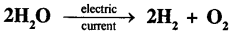(a)
Carbon with sulphurThe solid (products) changes to liquid (reactants) state.

(b)
Ammonia with hydrogen chlorideThe gas (products) changes to solid (reactants) state.

(c)
Hydrogen with oxygen
2H2[ g] + O2[g] + 2H2O[l]

The gas (products) changes to liquid (reactants) state.

(d)
Decomposition of waterThe liquid (products) changes to gas (reactants) state.

Question 6.
In certain reactions a change of smell is observed. Give two examples from daily life of the same.
In certain chemical reactions – a change of smell is seen to be observed.

1. A fresh egg gets spoiled and turns into a rotten egg, or fresh food on keeping for a long time gets spoiled. Thus a chemical change may have undergone, which many result in a change in odour [foul smell maybe Evolved].
2. A piece of fresh bread is kept in the open for a few days. A foul smell maybe observed along with growth of fungus, after keeping the bread for a prolonged time.

Question 7.
In certain reactions an insoluble solid called precipitate is formed. State the colour and name of the precipitate formed in each of the following reactions involving addition of:
(a) Dilute hydrochloric acid to silver nitrate.
(b) Iron [II] sulphate to sodium hydroxide.
(c) Iron [III] chloride to ammonium hydroxide.
(d) Copper [II] sulphate to sodium hydroxide.
(e) Lead nitrate to ammonium hydroxide.?
(a)
In the reaction of Silver nitrate and dil. hydrochloric acidThe precipitate formed is Silver Chloride which is milky white in colour.

(b)
Iron [II] sulphate and sodium hydroxideThe precipitate formed is Iron [II] hydroxide which is dirty green in colour.

(c)
Iron [III] chloride and ammonium hydroxide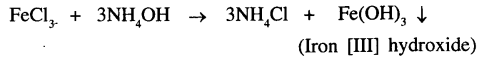The precipitate formed is Iron [III] hydroxide which is reddish brown in colour.

(d)
Copper [II] sulphate and sodium hydroxide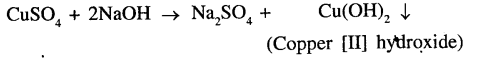The precipitate formed is Copper [II] hydroxide which is pale blue in colour.

(e)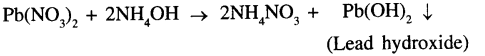The precipitate formed is Lead hydroxide which is chalky white in colour.

Question 8.
Give examples of exothermic reactions i.e. heat evolved in each of the following:
(a) reaction between a solid and a liquid
(b) reaction between two gases
Give two examples of exothermic reactions seen in everyday life.
(a)
Reaction between a solid and a liquid:

CaO + H2Ca(OH)3 + Δ

(b)
Reaction between two gases are: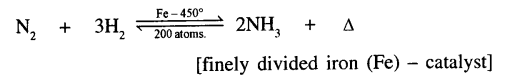Examples of exothermic reactions are:

1. Rusting of iron – the reaction is slow and the heat evolved is very less, hence unnoticeable.
2. Setting of cement – cement setting liberates heat, hence concrete structures are set slowly.
3. Human Body – many chemical reactions in our body are exothermic & provide energy to survive.

Question 9.
Give one example in each case where supplying energy [given below] is necessary for a chemical reaction.
(a) Heat energy
(b) Light energy
(c) Electrical energy
(d) Pressure
(e) Catalyst
(a)
Heat Energy:
On heating the reactants – the reacting particles, collide swiftly and with greater intensity.
Example:
Heat on zinc carbonate:(b)
Light Energy:
In presence of light – the molecules of the reactants absorb light energy and collide swiftly and react rapidly.
Example:
Photosynthesis in plants:
Plants manufacture food [glucose] from carbon dioxide and water – in the presence of chlorophyll [green pigment in plants] and sunlight.(c)
Electrical Energy:
It dissociates the reactant in molten or solution state, into ions, which are charged particles and hence respective products are obtained.
Example:
Electrolysis of acidified water.(d)
Pressure:
It causes the reacting molecules, to come together and collide with greater intensity.
Example:
Production of ammonia.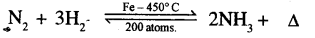(e)
Catalyst:
It is a substance which alters the rate of the reaction [i.e. increases or decreases the rate] without taking part in the reaction.It itself remains chemically unchanged at the end of the reaction.
Example: Catalytic oxidation of ammonia – using catalyst platinum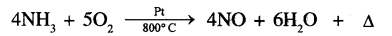Question 10.
Representation of the results of a chemical change – is a chemical equation.
For the equation: FeCl3 + 3NH4OH 3NH4Cl + Fe(OH)3
(a) Write the word equation for the above molecular equation.
(b) Is the equation given above a balanced equation. Give reasons.
(c) Name the reactants and the products in the above equation.
(d) What is the indications of the arrow between the reactants and the products and of the arrow pointing downwards at the end.
(a)
Iron (III) chloride + ammonium hydroxide → Ammonium chloride + Iron (III) hydroxide
(b)
Yes, because in this reaction the total number of atoms of each elements in the reactants, on the left side of the equation is equal to the number of products formed on the right side of the equation.
(c)
Reactants:
Iron (III) chloride and ammonium hydroxide (i.e. FeCl3 and – 3NH4OH)
Products:
Ammonium Chloride and Iron (III) chloride (i.e. 3NH4Cl and Fe (OH3)]
(d)

1. The function of the arrow(→)between the reactants & products is that it represents the chemical reaction taken place between two reactants.
2. Arrow pointing downwards (↓) at the end shows the precipitate formed in the chemical equation.

Question 11.
Give word equations for the following chemical reactions and give the names of the products formed.
(a) Zn + 2HCl→ ZnCl2 + H2
(b) CuO + H2SO4→CuSO4 + H2O
(c) Zn + S → ZnS
(d) ZnCO3 → ZnO + CO2
(e) NH3 + HCl→ NH4Cl
(a)
Zinc + dilute Hydrochloric acid→ Zinc chloride + Hydrogen
The product formed is gas evolved i.e. hydrogen.
(b)
Copper + Sulphuric → Copper(II)sulphate + water oxide acid
oxide acid
The product formed is Water (H20).
(c)
Zinc + Sulphur → Zinc Sulphide
The product formed is Zinc Sulphide (ZnS.
(d)
Zinc carbonate →Zinc oxide + Carbon dioxide
The product formed is gas evolved i.e. Carbon dioxide.
(e)
Ammonia + Hydrogen chloride → Ammonium chloride The product formed is Ammonium Chloride.

Objective Type Questions

1. Select the correct answer from A, B, C, D and E for each statement given below:

A: Hydrogen B: Copper [II] oxide C: Oxygen D: Copper [II] hydroxide E: Ammonia

1. The black residue obtained on heating copper carbonate.
Ans. B: Copper [II] oxide
2. The basic gas obtained when two gases react in presence of a catalyst and the reaction is exothermic.
Ans. A: Hydrogen
3. The gas obtained when potassium chlorate is heated in the presence of a catalyst.
Ans. C: Oxygen
4. The pale blue precipitated obtained when copper [II] sulphate reacts with sodium hydroxide.
Ans. D: Copper [II] hydroxide
5. The gas which reacts with oxygen to give a neutral liquid.
Ans. E: Ammonia

2. Give a word equation for each of the following reactions.

Question 1.
Two solids which combine on heating, to give a liquid.
Carbon + Sulphur → Carbon disulphide
C + S → CS2 (l)

Question 2.
Two gases which combine to give a solid.
Ammonia + Hydrogen chloride→ Ammonium chloride
NH3 (g) + HCl (g)→NH4Cl (s)

Question 3.
A compound which on heating shows – colour change from brown to yellow.
2Pb02 →2PbO + 02 (l)

Question 4.
A salt which reacts with ammonium hydroxide to give a reddish brown precipitate.
Iron (III) Chloride + ammonium hydroxide → Ammonium Chloride + Iron (III) hydroxide
FeCl3 + 3NH4OH → 3NH4Cl + Fe(OH)2

Question 5.
An exothermic reaction where both the reactants are gases, one of which is oxygen.
Hydrogen + Oxygen → water
2H2(g) + O2(g) → H2O(l)

3. Name the following:

Question 1.
The silvery residue obtained on heating mercury [II] oxide.
The silvery residue obtained on heating Mercury [II] oxide is Mercury (Hg).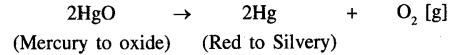Question 2.
The gas evolved when a dilute acid is added to chalk [limestone].
Carbon dioxide (CO2)

Question 3.
Two non-metal which react explosively when brought in close contact.
Iodine and phosphorous

Question 4.
Two gases which react under pressure in presence of a catalyst at elevated temperatures to give a gaseous product.
Nitrogen and Hydrogen

Question 5.
A catalyst which increases the rate of a chemical reaction.
Manganese dioxide (MnO2).

4.Give reasons for the following:

Question 1.
Even though rusting is an exothermic reaction, the heat evolved when an iron nail rusts is unnoticeable.
Rusting of iron – the reaction is slow and the heat evolved is very less, hence unnoticeable.

Question 2.
Electrolytic decomposition of water is a reaction involving change of state.
Electrolysis of water is the decomposition of water into hydrogen and oxygen due to the passage of electric current through it.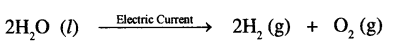Question 3.
A characteristic change of colour is seen when a piece of iron is added to copper [II] sulphate solution.
A characteristic change of colour is seen when piece of iron is added to Copper (II) sulphate Solution. This is because iron displaces copper from the copper sulphate solution and forming ferrous sulphate
CuSO4 + Fe → FeSO4 + Cu.
Hence, Blue colour of the solution in changes to pale green and there is a reddish brown deposits on copper.

Question 4.
The catalytic oxidation of sulphur dioxide to sulphur trioxide is represented by a double arrow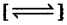between the reactants and products, formed in the chemical reaction.
The reaction is reversible shown by the double arrow.
The Equation of Oxidation of sulphur dioxide to sulphur trioxide is as follows: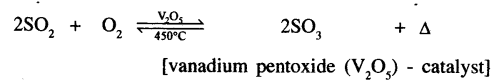Question 5.
Silver salts are kept in dark coloured bottles.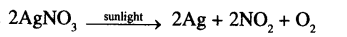Silver nitrate gets decomposed by sunlight. Hence silver nitrate is stored in coloured reagent bottles.

5. Write word equations for the following chemical reactions given below. Also state the observation seen in each case.
Ans:
Question 1.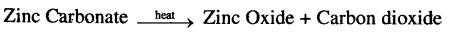CO2 (g) is formed in the above chemical reaction.

Question 2.
NaCl + AgNO3 AgCU ↓+ NaNO3
Sodium Chloride + Silver nitrate → Silver chloride + Sodium nitrate
AgCl (Silver Chloride) a white precipitate is formed in the above chemical reaction.

Question 3.Sodium sulphite + Hydrochloric acid → Sodium chloride + water + Sulphur dioxide
S02 (g) Sulphur dioxide is formed in the above chemical reaction.

Question 4.